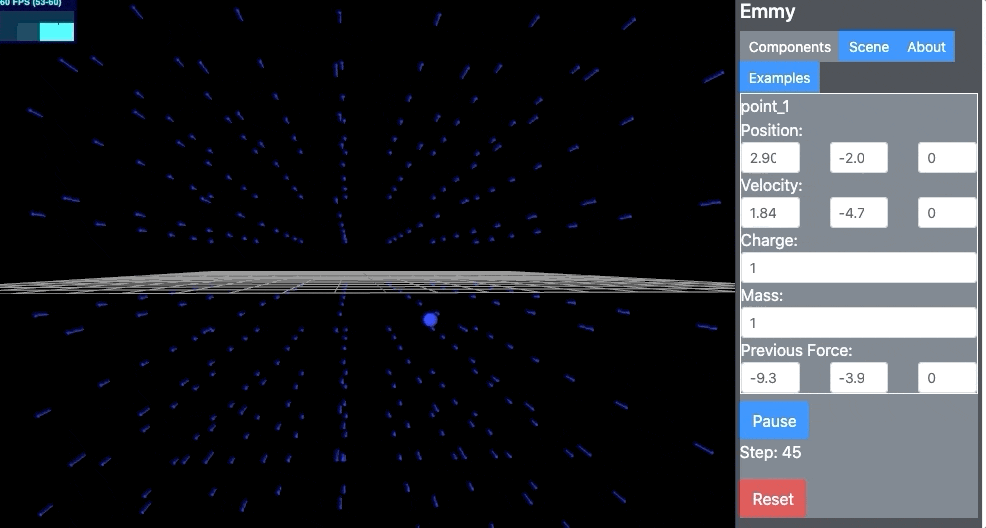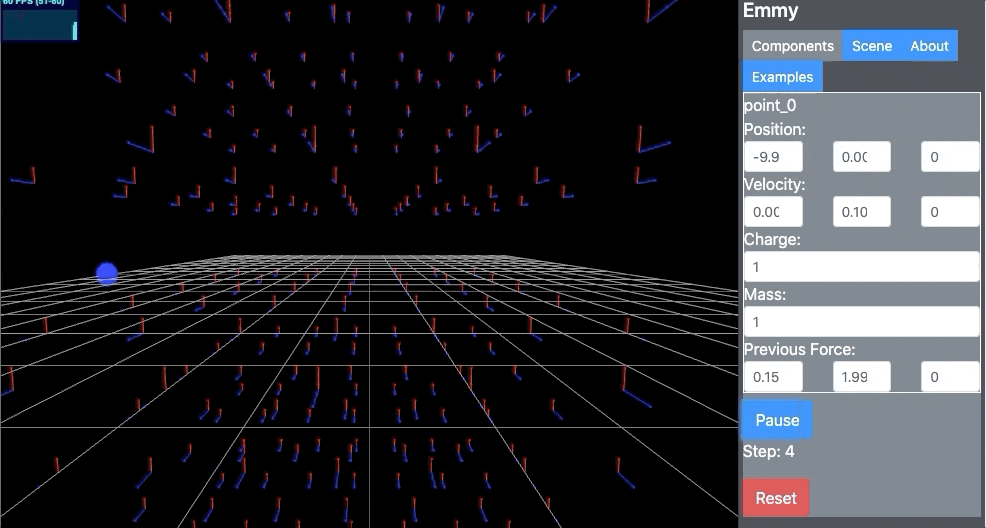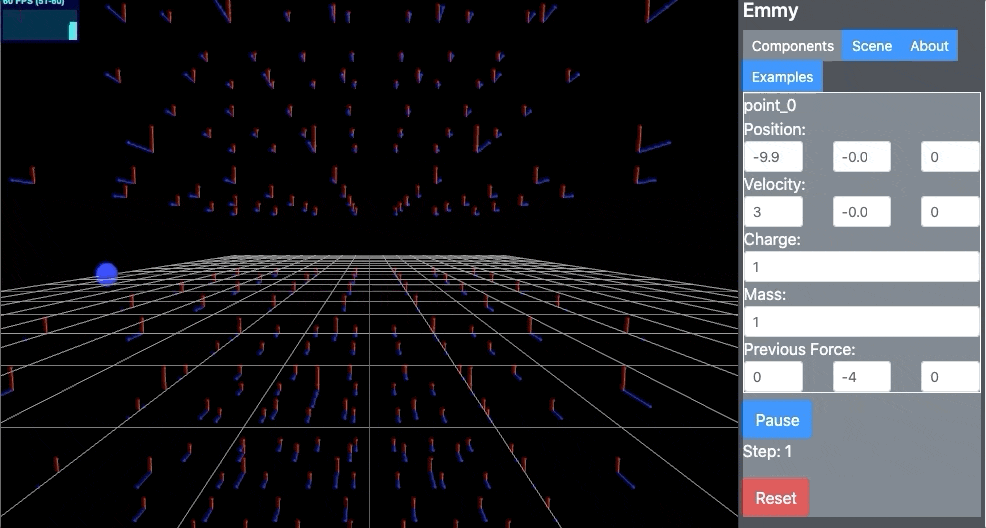# EM Particle Motion

Simulating a particle under the influence. (Of electric and magnetic fields).

## Introduction

Basically this is just a simulation of Lorentz Force law:

$$\bm{F} = q(\bm{E} + \bm{r} \times \bm{B} )$$

What’s fun is that if you place a charge in these Electric and Magnetic field equations, the particle takes a pretty peculiar path!

## Results

Check it out here! Although, I kind of rushed through this, so I think I broke some of the other examples. You can click pause and change the velocity of the particle.

https://emmy-viz.github.io/emmy/

### Code

I used emmy for this project, so the code was super simple:

sim_charged.addPoint(new Vector(-10, 0, 0), 1)
sim_charged.e_fields.push(new VectorField(new Func("0"), new Func("2"), new Func("0")))
sim_charged.b_fields.push(new VectorField(new Func("0"), new Func("0"), new Func("2")))


The code adds a point charge with q=1, then adds $$\bm{E} = 2\hat{y}$$ and $$\bm{B} = 2\hat{z}$$.

Technically emmy didn’t have support for magnetic fields, but that was pretty quick to add. Within the simulator there’s a step function that uses implicit euler as the integrator, so I just added the b-field force to the existing force equation.

### B field, $$v_x = 0$$

So a particle under the force of a B-Field with no initial velocity:It’s moves in a circle!

### B & E field, $$v_x = 0$$

If we add an E field, things get weird. It bounces up and down along the axis.

That is not what I would have guessed the motion would be.### B & E field, $$v_x = 3$$$$v_x = 3$$ is to the right along the line it’s currently on.

It’s neat that the particle stays below the plane. Because in the next one…

### B & E field, $$v_x = -1$$

This particle moves above the plane! And it’s interesting to note I “pushed” it with an initial velocity of -1, but the E and B field said no no and moved it to the right.### B & E field, $$v_x = 2$$

When v=2, the particle pinches at the bottom of it’s motion, and then goes back up.## What went well?

• I’m happy I setup Makefiles for all my projects. Having “make run” and “make deploy” for each projects makes development so much easier.

## What did not go well?

• I quickly copied some code (specifically the code for drawing vectorfields), and I didn’t change all the “e_field” to “b_field” and had a weird issue to debug. It ended up being two different issues in one. (the vectorfields were returning NaN).

## What’s next?

Nothing for this project. I might clean up emmy in the future though. It’s cool that adding magnetic fields was so easy.

I’d really like to move to relatavistic EM tensors. But, probably not going to do that anytime soon.